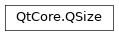# QSize¶

The `QSize` class defines the size of a two-dimensional object using integer point precision. More## Detailed Description¶

A size is specified by a `width()` and a `height()` . It can be set in the constructor and changed using the `setWidth()` , `setHeight()` , or `scale()` functions, or using arithmetic operators. A size can also be manipulated directly by retrieving references to the width and height using the `rwidth()` and `rheight()` functions. Finally, the width and height can be swapped using the `transpose()` function.

The `isValid()` function determines if a size is valid (a valid size has both width and height greater than or equal to zero). The `isEmpty()` function returns `true` if either of the width and height is less than, or equal to, zero, while the `isNull()` function returns `true` only if both the width and the height is zero.

Use the `expandedTo()` function to retrieve a size which holds the maximum height and width of this size and a given size. Similarly, the `boundedTo()` function returns a size which holds the minimum height and width of this size and a given size.

`QSize` objects can be streamed as well as compared.

class PySide2.QtCore.QSize

PySide2.QtCore.QSize(QSize)

PySide2.QtCore.QSize(w, h)

param w:

int

param QSize:

`PySide2.QtCore.QSize`

param h:

int

Constructs a size with an invalid width and height (i.e., `isValid()` returns `false` ).

Constructs a size with the given `width` and `height` .

PySide2.QtCore.QSize.__reduce__()
Return type:

object

PySide2.QtCore.QSize.__repr__()
Return type:

object

PySide2.QtCore.QSize.boundedTo(arg__1)
Parameters:
Return type:

`PySide2.QtCore.QSize`

Returns a size holding the minimum width and height of this size and the given `otherSize` .

PySide2.QtCore.QSize.expandedTo(arg__1)
Parameters:
Return type:

`PySide2.QtCore.QSize`

Returns a size holding the maximum width and height of this size and the given `otherSize` .

PySide2.QtCore.QSize.grownBy(m)
Parameters:
Return type:

`PySide2.QtCore.QSize`

PySide2.QtCore.QSize.height()
Return type:

int

Returns the height.

PySide2.QtCore.QSize.isEmpty()
Return type:

bool

Returns `true` if either of the width and height is less than or equal to 0; otherwise returns `false` .

PySide2.QtCore.QSize.isNull()
Return type:

bool

Returns `true` if both the width and height is 0; otherwise returns false.

PySide2.QtCore.QSize.isValid()
Return type:

bool

Returns `true` if both the width and height is equal to or greater than 0; otherwise returns `false` .

PySide2.QtCore.QSize.__ne__(s2)
Parameters:
Return type:

bool

PySide2.QtCore.QSize.__mul__(c)
Parameters:

c – float

Return type:

`PySide2.QtCore.QSize`

PySide2.QtCore.QSize.__mul__(c)
Parameters:

c – float

Return type:

`PySide2.QtCore.QSize`

PySide2.QtCore.QSize.__imul__(c)
Parameters:

c – float

Return type:

`PySide2.QtCore.QSize`

Multiplies both the width and height by the given `factor` , and returns a reference to the size.

Note that the result is rounded to the nearest integer.

Parameters:
Return type:

`PySide2.QtCore.QSize`

Parameters:
Return type:

`PySide2.QtCore.QSize`

Adds the given `size` to this size, and returns a reference to this size. For example:

```s = QSize( 3, 7)
r = QSize(-1, 4)
s += r

# s becomes (2,11)
```
PySide2.QtCore.QSize.__sub__(s2)
Parameters:
Return type:

`PySide2.QtCore.QSize`

PySide2.QtCore.QSize.__isub__(arg__1)
Parameters:
Return type:

`PySide2.QtCore.QSize`

Subtracts the given `size` from this size, and returns a reference to this size. For example:

```s = QSize( 3, 7)
r = QSize(-1, 4)
s -= r

# s becomes (4,3)
```
PySide2.QtCore.QSize.__div__(c)
Parameters:

c – float

Return type:

`PySide2.QtCore.QSize`

PySide2.QtCore.QSize.__idiv__(c)
Parameters:

c – float

Return type:

`PySide2.QtCore.QSize`

Divides both the width and height by the given `divisor` , and returns a reference to the size.

Note that the result is rounded to the nearest integer.

PySide2.QtCore.QSize.__eq__(s2)
Parameters:
Return type:

bool

PySide2.QtCore.QSize.scale(s, mode)
Parameters:

Scales the size to a rectangle with the given `size` , according to the specified `mode` .

PySide2.QtCore.QSize.scale(w, h, mode)
Parameters:
• w – int

• h – int

Scales the size to a rectangle with the given `width` and `height` , according to the specified `mode` :

• If `mode` is `IgnoreAspectRatio` , the size is set to (`width`, `height`).

• If `mode` is `KeepAspectRatio` , the current size is scaled to a rectangle as large as possible inside (`width`, `height`), preserving the aspect ratio.

• If `mode` is `KeepAspectRatioByExpanding` , the current size is scaled to a rectangle as small as possible outside (`width`, `height`), preserving the aspect ratio.

Example:

```t1 = QSize(10, 12)
t1.scale(60, 60, Qt.IgnoreAspectRatio)
# t1 is (60, 60)

t2 = QSize(10, 12)
t2.scale(60, 60, Qt.KeepAspectRatio)
# t2 is (50, 60)

t3 = QSize(10, 12)
t3.scale(60, 60, Qt.KeepAspectRatioByExpanding)
# t3 is (60, 72)
```
PySide2.QtCore.QSize.scaled(s, mode)
Parameters:
Return type:

`PySide2.QtCore.QSize`

Return a size scaled to a rectangle with the given size `s` , according to the specified `mode` .

PySide2.QtCore.QSize.scaled(w, h, mode)
Parameters:
• w – int

• h – int

Return type:

`PySide2.QtCore.QSize`

Return a size scaled to a rectangle with the given `width` and `height` , according to the specified `mode` .

PySide2.QtCore.QSize.setHeight(h)
Parameters:

h – int

Sets the height to the given `height` .

`rheight()` `height()` `setWidth()`

PySide2.QtCore.QSize.setWidth(w)
Parameters:

w – int

Sets the width to the given `width` .

`rwidth()` `width()` `setHeight()`

PySide2.QtCore.QSize.shrunkBy(m)
Parameters:
Return type:

`PySide2.QtCore.QSize`

PySide2.QtCore.QSize.toTuple()
Return type:

object

PySide2.QtCore.QSize.transpose()

Swaps the width and height values.

PySide2.QtCore.QSize.transposed()
Return type:

`PySide2.QtCore.QSize`

Returns a `QSize` with width and height swapped.

PySide2.QtCore.QSize.width()
Return type:

int

Returns the width.